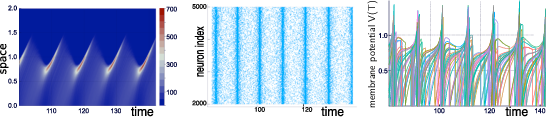EN FR
EN FR
##### MATHNEURO - 2018
Partnerships and Cooperations
Bibliography
Partnerships and Cooperations
Bibliography

## Section: Research Program

### Mean-field and stochastic approaches

Modeling neural activity at scales integrating the effect of thousands of neurons is of central importance for several reasons. First, most imaging techniques are not able to measure individual neuron activity (microscopic scale), but are instead measuring mesoscopic effects resulting from the activity of several hundreds to several hundreds of thousands of neurons. Second, anatomical data recorded in the cortex reveal the existence of structures, such as the cortical columns, with a diameter of about 50$\mu m$ to 1$mm$, containing of the order of one hundred to one hundred thousand neurons belonging to a few different species. The description of this collective dynamics requires models which are different from individual neurons models. In particular, when the number of neurons is large enough averaging effects appear, and the collective dynamics is well described by an effective mean-field, summarizing the effect of the interactions of a neuron with the other neurons, and depending on a few effective control parameters. This vision, inherited from statistical physics requires that the space scale be large enough to include a large number of microscopic components (here neurons) and small enough so that the region considered is homogeneous.

Our group is developing mathematical and numerical methods allowing on one hand to produce dynamic mean-field equations from the physiological characteristics of neural structure (neurons type, synapse type and anatomical connectivity between neurons populations), and on the other so simulate these equations; see Figure 1. These methods use tools from advanced probability theory such as the theory of Large Deviations  and the study of interacting diffusions .

Figure 1. Simulations of the quasi-synchronous state of a stochastic neural network with $N=5000$ neurons. Left: empirical distribution of membrane potential as a function $\left(t,v\right)$. Middle: (raster plot) spiking times as a function of neuron index and time. Right: several membrane potentials ${v}_{i}\left(t\right)$ as a function of time for $i\in \left[1,100\right]$. Simulated with the Julia Package PDMP.jl from . This figure has been slightly modified from .